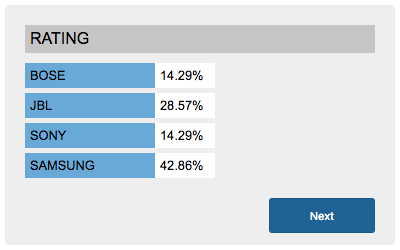# PHP Poll Script

In this tutorial, we are going to learn how to create a poll script for rating using PHP. In a previous tutorial, we have seen PHP star rating using jQuery. In this example poll script, there are questions with multiple options to add user rating in a poll. After submitting the poll rating, the overall rating percentage for all the options will be shown to the users.

In this script, I have used jQuery AJAX to show set poll options and save user poll options into the backend database tables. The user session id is defined in the beginning and the poll rating is saved into the database for the user session. On clicking next, the next unrated question will be shown to the user.

View Demo## Set Poll Options to Add Rating

In this section, we are going to read questions and poll options from database to show them for user rating. I send a AJAX call to the PHP script to get a question which is not yet rated by the user. This script will respond the poll HTML to the user to submit their rating. The jQuery AJAX and the PHP script is shown below.

```function show_poll(){
\$.ajax({
type: "POST",
url: "show-poll.php",
processData : false,
beforeSend: function() {
\$("#overlay").show();
},
success: function(responseHTML){
\$("#overlay").hide();
\$("#poll-content").html(responseHTML);
}
});
}
```

### show-poll.php

```<?php
session_start();
\$_SESSION["member_id"] = 1;

require("dbcontroller.php");
\$dbController = new DBController();

\$query = "SELECT DISTINCT question_id from tbl_poll WHERE member_id = " . \$_SESSION["member_id"];
\$result = \$dbController->getIds(\$query);

\$condition = "";
if(!empty(\$result)) {
\$condition = " WHERE id NOT IN (" . implode(",", \$result) . ")";
}

\$query = "SELECT * FROM `tbl_question` " . \$condition . " limit 1";
\$questions = \$dbController->runQuery(\$query);

if(!empty(\$questions)) {
?>
<div class="question"><?php echo \$questions["question"]; ?><input type="hidden" name="question" id="question" value="<?php echo \$questions["id"]; ?>" ></div>
<?php
\$query = "SELECT * FROM tbl_answer WHERE question_id = " . \$questions["id"];
?>
<?php
}
}
?>
<div class="poll-bottom">
</div>
<?php
} else {
?>
<div class='error'>No Questions Found</div>
<?php
}
?>
```

## Save Rating using jQuery AJAX

In this section, I receive the user rating and send it to the PHP script to update rating database. On submitting the poll rating it invokes jQuery AJAX function to request PHP to update rating. After updating rating table, the response will show the overall rating in percentage. The PHP and AJAX code is,

```function addPoll() {
\$.ajax({
type: "POST",
url: "save-poll.php",
processData : false,
beforeSend: function() {
\$("#overlay").show();
},
success: function(responseHTML){
\$("#overlay").hide();
\$("#poll-content").html(responseHTML);
}
});

}
}
```

### save-poll.php

```<?php
session_start();
require("dbcontroller.php");
\$dbController = new DBController();

\$question_id = \$_POST['question'];

\$query = "INSERT INTO tbl_poll(question_id,answer_id,member_id) VALUES ('" . \$question_id ."','" . \$answer_id . "','" . \$_SESSION["member_id"] . "')";
\$insert_id = \$dbController->insertQuery(\$query);

if(!empty(\$insert_id)) {
\$query = "SELECT * FROM tbl_answer WHERE question_id = " . \$question_id;
}

?>
<?php
\$query = "SELECT count(answer_id) as total_count FROM tbl_poll WHERE question_id = " . \$question_id;
\$total_rating = \$dbController->runQuery(\$query);

}
\$percentage = 0;
if(!empty(\$total_rating)) {
\$percentage = ( \$answers_count / \$total_rating["total_count"] ) * 100;
if(is_float(\$percentage)) {
\$percentage = number_format(\$percentage,2);
}
}

?>
<?php
}
}
?>
<div class="poll-bottom">